# Uncertainty in Physical Measurements Module 1 - Excel Version

Uncertainty Module 1 - UM Student Guide May 15, 2016, 2:44 p.m.

## Backgammon 101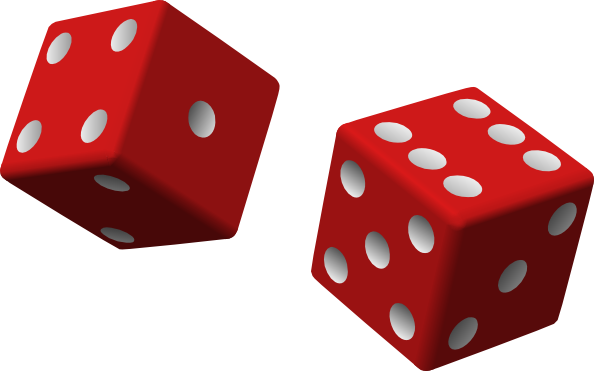In this Module, we will consider dice. Although people have been gambling with dice and related apparatus since at least 3500 BCE, amazingly it was not until the mid-sixteenth century that Cardano began to discover the statistics of dice that we will think about here.

Although you will not be doing a huge amount of calculation as you go through this Module, you should use Microsoft Excel for both the calculations and making the histogram. If you do not know how to use Excel now is a good time to begin learning this important tool. The Appendix has some tips on using Excel for people who have some knowledge of the software, and some links to videos for beginners. After completing this Module, you will want to print the Excel worksheet and staple it into your notebook.

For an honest die with an honest roll, each of the six faces are equally likely to be facing up after the throw. Thus, for a pair of dice there are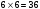equally likely combinations. Of these combinations there is only one, 1-1 (“snake eyes”), whose sum is 2. Thus the probability of rolling a two is 1/36 = 0.03 = 3%. Similarly, there are two combinations whose sum is 3, 1-2 and 2-1, so the probability of rolling a three is 2/36. Table 1 summarises all of the possible combinations.

 Probabilities for honest dice Sum Combinations Number Probability 2 1-1 1 1/36 = 0.03 3 1-2, 2-1 2 2/36 = 0.06 4 1-3, 3-1, 2-2 3 3/36 = 0.08 5 2-3, 3-2, 1-4, 4-1 4 4/36 = 0.11 6 2-4, 4-2, 1-5, 5-1, 3-3 5 5/36 = 0.14 7 3-4, 4-3, 2-5, 5-2, 1-6, 6-1 6 6/36 = 0.17 8 3-5, 5-3, 2-6, 6-2, 4-4 5 5/36 = 0.14 9 3-6, 6-3, 4-5, 5-4 4 4/36 = 0.11 10 4-6, 6-4, 5-5 3 3/36 = 0.08 11 5-6, 6-5 2 2/36 = 0.06 12 6-6 1 1/36 = 0.03

#### Table 1

A histogram is a convenient way to display numerical results. You have probably seen histograms of grade distributions on a test. If we roll a pair of dice 36 times and the results exactly match the above theoretical prediction, then a histogram of the results would look like the following: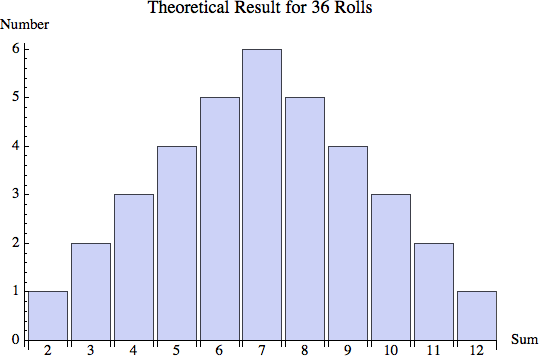##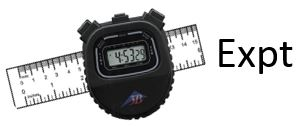Activity 1

Roll the supplied pair of dice 36 times, recording each result. Make a histogram of the results. Qualitatively how do your results of this experiment compare to the theoretical prediction?

Although this Module is part of a series on Uncertainty in Physical Measurements, the result of throwing the dice one time is certain: it is definitely a particular number. However, there is uncertainty associated with predicting the result of throwing the dice before you actually do it.

Your Instructors will collect the data for all the Teams’ results and combine them into a single dataset and histogram which will be shown on the screen later in this Module.

##Questions

1. What is the probability of rolling a seven 10 times in a row?

2. Amazingly, you have rolled a seven 9 times in a row. What is the probability that the tenth roll will also come up with a seven?

A particularly powerful way of visualising the results shown Table 1 is to show the probability as a function of the sum. This is called a probability distribution, as shown in Figure 2. You will want to note that in the figure:

• We have added two “free” data points for sums of 1 and 13. Both of these are impossible, so their probabilities are 0.

• We have connected the dots.

• We have indicated the distance a from the maximum to the where the probability is 0 on the right. The quantity a is called the half-width of the distribution.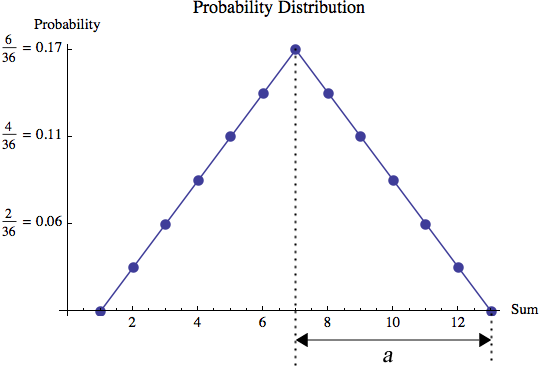#### Figure 2

The line connecting the dots defines the probability distribution function (pdf) for rolling dice. This probability distribution function is triangular. We can write the pdf as:

$$pdf{\rm (Sum)} = \begin{cases} \frac{1}{36}({\rm Sum}-1), & 1 \leq {\rm Sum} \leq 7 \\ \frac{1}{36}(13-{\rm Sum}), & 7 < {\rm Sum} \leq 13 \\ 0, & {\rm Otherwise} \end{cases}$$          (1)

We will see other examples of triangular probability distributions later, and will also learn about other shapes for probability distributions.

##Questions

1. What is the sum of all of the probabilities given in Table 1? Explain why this result is reasonable.

2. Imagine that you are about to roll the dice. What is the probability that the result will be 5, 6, 7, 8, or 9?

3. What is the total area under the pdf ? Although integral calculus can find this solution using Eqn. 1, it is perfectly acceptable to answer this question using the fact that the area of a triangle is $$\frac{1}{2} \times base \times height$$. How does your result compare to your answer to Question 3?

In your experiment you have collected 36 values of the result of throwing the dice. We will call each individual result Si where i is an integer between 1 and 36. Then the mean or average of the 36 values is given the symbol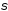and is given by:

$$\begin{eqnarray*} \bar{S}=\frac{\sum\limits^{36}_{i=1}S_i}{36} \end{eqnarray*}$$          (2)

For a symmetric probability distribution function, such as the triangular pdf that describes the dice, the theoretical value of the mean is the midpoint of the pdf, which in this case is 7.

Each individual measurement Si may differ from the mean. The deviation di of each measurement from the mean is:

$$d_i \equiv S_i - \bar{S}$$            (3)

##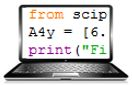Activity 2

Calculate the mean of your experimental results. Qualitatively, is this close to the theoretical prediction of 7?

Calculate the deviations of each of your individual measurements.

What the sum of the 36 deviations? Is this a reasonable result? Why?

In Activity 2, you have seen that the sum of the deviations is not very useful. However, the sum of the square of the deviations does provide information on how your closely measurements are to the mean value. However, the value of the sum of the squares of the deviations depends on how many measurements were made. The standard way of dealing with this is the variance var, which normalises the sum by the number of measurements N – 1. The variance is defined as:

$$\begin{eqnarray*} var\equiv \frac{\sum\limits^{N}_{i=1}(d_i)^2}{N-1} \end{eqnarray*}$$​       (4)

In general, for a triangular probability distribution function with data which matches the theoretical prediction, the variance can be shown to be:

$$\begin{eqnarray*} var = \frac{a^2}{6} \end{eqnarray*}$$        (5)

For the triangular probability distribution function of Figure 2, a = 6 and the variance is 6. Although we are not asking you to do so, it is fairly simple to show that this is correct for the data of Table 1.

You may wish to note that the definition of the variance, Eqn. 4, means that it can not be calculated for one measurement, since if N = 1 the denominator is zero: this is completely reasonable. Also for a large number of measurements the denominator  $$N - 1 \approx N$$and the variance is approximately the mean of the deviations squared.

##Questions

1. Three different Teams, A, B, and C, have done the same experiment that you did by rolling the dice 36 times. The mean of their values to 3 significant figures were all 7.17. Here are histograms of their results: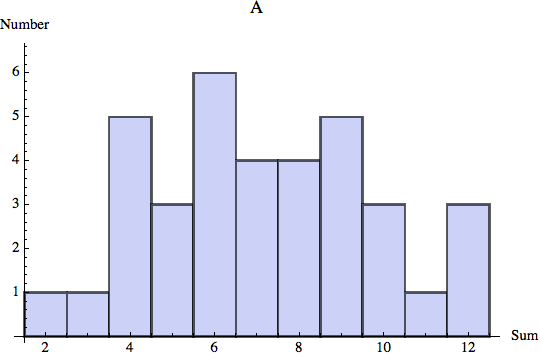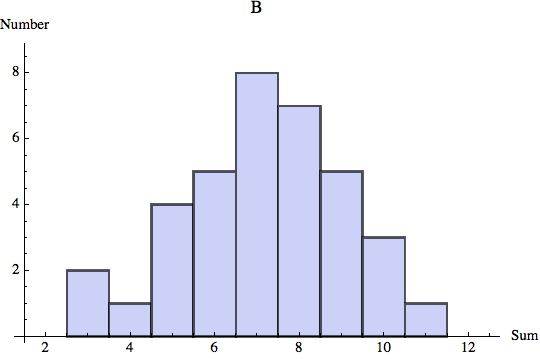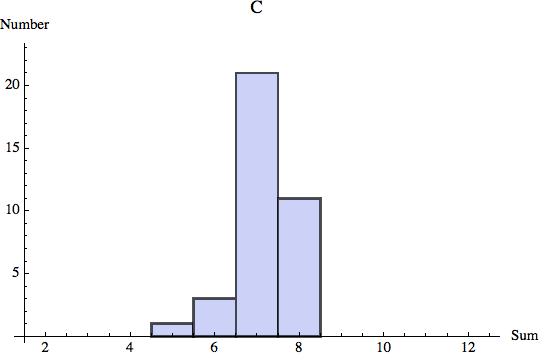Rank the variances of these three experiments. You do not need to do any calculations to do the ranking.

1. What is the variance of your experimental data?

##Activity 3

Do the combined results for all Teams, collected and shown by your Instructors, compare better or worse to the theoretical prediction than your experimental result for 36 rolls of the dice? Why do you think this is so?

Devise a numerical method to define the phrase “close to the theoretical result.” Note that there is no single correct answer to this. Some possible ways to do this is that if you roll $$Sum_{4, {\rm expt}}$$ fours while the theoretical prediction of number of fours is ​$$Sum_{4, {\rm theory}}$$​ , then you can define “close” to be that the absolute value of the difference is less than or equal to some number N, i.e. $$|Sum_{4,{\rm expt}}-Sum_{4,{\rm theory}}| \leq N$$ . Or perhaps you decide it is more reasonable to define “close” to be that the difference is less than or equal to some fraction f of the theoretical value:  ​$$|Sum_{4,{\rm expt}}-Sum_{4,{\rm theory}}| \leq f\ Sum_{4,{\rm theory}}$$​ . You can perhaps devise other reasonable methods. In Module 6 you will re-visit the definition of “close”.

Use your method to quantitatively express how well your experiment’s results compare to the theoretical prediction, and how well the overall combined results compare to the theoretical prediction.

What would be necessary for you to be able to guarantee that the result of an experiment of rolling dice conforms exactly to the theoretical prediction? Is this possible to actually do?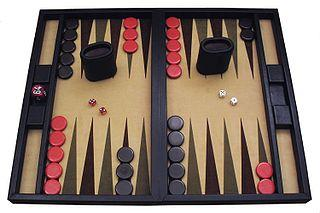Backgammon is a game in which the pieces are moved according to the roll of dice. Like poker, it is a game of skill disguised as a game of chance: in the long run the winning player is the one who best understands, at least intuitively, the ideas of probability we have explored above.

## Summary of Names, Symbols, and Formulae

Histogram: a graphical representation of the distribution of data. It is a series of rectangles whose height is proportional to the number of measurements in the range of values represented on the horizontal axis.

Probability Distribution: a distribution of probabilities.

Half-width a: one-half of the total width of a probability distribution.

Probability Distribution Function (pdf): a function representing a probability distribution.

For N repeated measurements: x1, x2, x3, … , xN:

Mean or average $$\begin{eqnarray*} \bar{x} = \frac{\sum\limits_{i=1}^N x_i}{N} \end{eqnarray*}$$

Deviation  $$d_i = x_i - \bar{x}$$

Variance$$\begin{eqnarray*} var\equiv \frac{\sum\limits^{N}_{i=1}(d_i)^2}{N-1} \end{eqnarray*}$$

For a triangular pdf of half-width a   $$\begin{eqnarray*} var = \frac{a^2}{6} \end{eqnarray*}$$

## Appendix

If you are already reasonably familiar with Excel then we will begin by giving you some tips on using it with this Module. For relative beginners, we have prepared some videos on using Excel for which links are provided below.

Here are the tips:

• Collect the raw data in column A. Labeling the column with some name like raw in the first row will make your workbook much easier to read. After each roll enter the sum of the two dice in the next empty cell in the column.
• When all 36 data points are entered, copy all of column A into column B. Change the label to some name like sorted.
• Sort the values in column B from rows 2 through 37 in ascending order.When prompted, do not expand the selection.
• Leave a few empty columns and in some column, say column F, construct the numbers that will be histogram.It will be convenient to explicitly enter a 0 into row 1.Then the total number of twos in the sorted column is entered into row 2, the total number of threes into row 3, etc. through row 12. Although you can make Excel fill out the values using its COUNT function, it is probably just as fast to manually count the number of twos, number of threes, etc.
• Select all the data in the column you have just filled out and make a Clustered Column chart. This is the histogram.You may wish to clean up the histogram, but it is not necessary.
• Somewhere to the right of the row with the histogram data, calculate the mean of the raw or sorted data using the AVERAGE function. Label the cell in an adjacent cell. Give the calculated value some name such as mean.
• You can then calculate the deviations in, say, column C, and the deviations squared in column D. Label the columns in row 1.
• You can calculate the variance directly from the deviations squared in column D or you can use the Excel VAR function.

As promised, here are links to the videos intended for Excel beginners. There are two videos:

The first video EntryHistogram.mp4 discusses how to enter the data and produce a histogram.

The second video AnalyseData.mp4 discusses how to analyse the data.

This Guide was written by David M. Harrison, Dept. of Physics, Univ. of Toronto, September 2013.